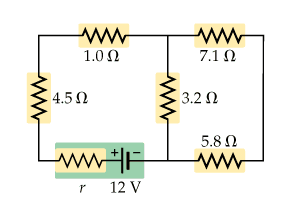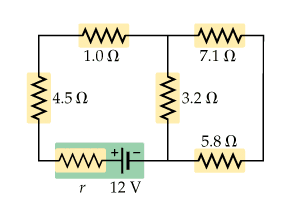# Calculate the equivalent resistance

## Homework StatementIgnore the internal resistance of the battery and assume it is merely a battery. Calculate the equivalent resistance of the circuit.

## Homework Equations

1/Req = 1/R + 1/R ... (parallel)
Req = R + R .... (series)

## The Attempt at a Solution

I know this is fairly simple but I dont understand how you would calculate the equivalent resistance for the three resistors (3.2 ohms, 7.1 ohms, 5.8 ohms). I assumed they were in series and simply added them, and then added 1.0 ohms and 4.5 ohms to get the total resistance. I think this is wrong, can someone explain how you would go about calculating the Req?

## Homework StatementIgnore the internal resistance of the battery and assume it is merely a battery. Calculate the equivalent resistance of the circuit.

## Homework Equations

1/Req = 1/R + 1/R ... (parallel)
Req = R + R .... (series)

## The Attempt at a Solution

I know this is fairly simple but I dont understand how you would calculate the equivalent resistance for the three resistors (3.2 ohms, 7.1 ohms, 5.8 ohms). I assumed they were in series and simply added them, and then added 1.0 ohms and 4.5 ohms to get the total resistance. I think this is wrong, can someone explain how you would go about calculating the Req?

I'm afraid you're wrong. All of them aren't in series. See the figure I've uploaded. What can you infer from that? Always deal with 2 resistances at a time. Don't involve 3 together.

#### Attachments

I see what you are saying, since 7.1 and 5.8 are in series, they must be added together? And then added in parallel to 3.2?

I see what you are saying, since 7.1 and 5.8 are in series, they must be added together? And then added in parallel to 3.2?
You got that right mate!

thanks!

thanks![/QUOT